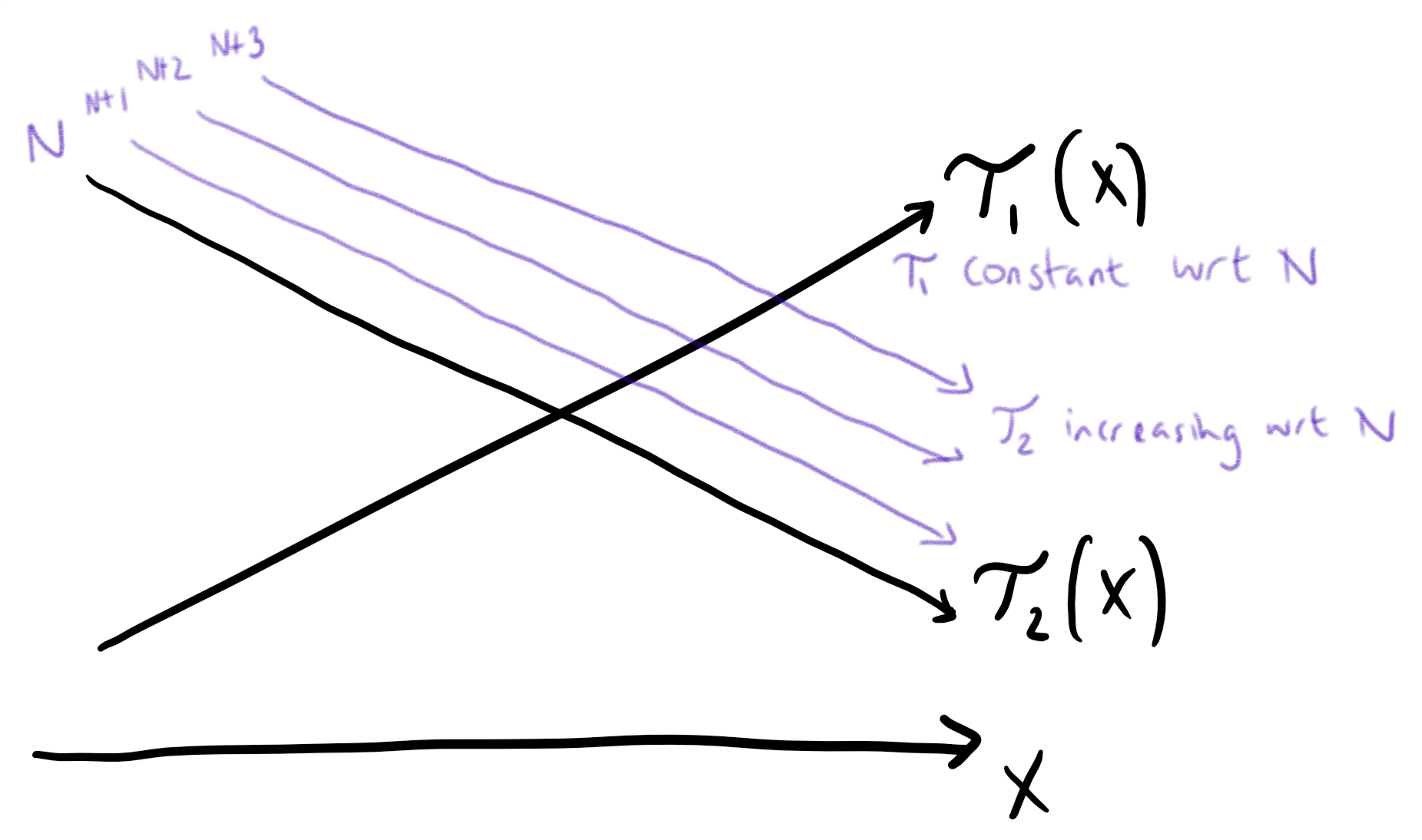‘Super Egg Drop’ is a very classic DP problem. Good articles explaining the O(KN*N) and the O(KN*logN) solutions can be easily found from the internet. Better than that, there is a solution of O(KN). However, there isn’t much about the O(KN) solution and the rare posts about it aren’t very clear. The article you are reading now is an endeavor to fill this gap.

# Check Out The Problem

You are given K eggs, and you have access to a building with N floors from 1 to N

Each egg is identical in function, and if an egg breaks, you cannot drop it again.

You know that there exists a floor F with 0 <= F <= N such that any egg dropped at a floor higher than F will break, and any egg dropped at or below floor F will not break.

Each move, you may take an egg (if you have an unbroken one) and drop it from any floor X (with 1 <= X <= N).

Your goal is to know with certainty what the value of F is.

What is the minimum number of moves that you need to know with certainty what F is, regardless of the initial value of F

##### Example:

Input: K = 1, N = 2
Output: 2
Explanation:
Drop the egg from floor 1. If it breaks, we know with certainty that F = 0.
Otherwise, drop the egg from floor 2. If it breaks, we know with certainty that F = 1.
If it didn’t break, then we know with certainty F = 2.
Hence, we needed 2 moves in the worst case to know what F is with certainty.

# Analysis

Firstly Let’s define dp(i,j) as the maximum number of floors we can surely find F from with i moves and j eggs. With this definition, the original question can be transformed into finding the minimum m whose dp(m,K) is larger than or equal to N.

Secondly, it can be easily observed that the maximum number of floors we can find F from is equal to the number of moves we can take if there is only 1 egg. In a formula, dp(i,1)=i.

Assuming that to get dp(m,K), the first floor where we drop the egg is dp(m-1, K-1)+1 floor (We will explain why choose this floor later). With this assumption in mind, there will be two different cases to analyse:

• #### The first egg breaks

From the breaking of the first egg, we know that the elusive F must be within first dp(m-1,K-1) floors. And with the poor broken first egg lost and the first move taken, we now can move m-1 times with K-1 eggs which is just enough for us to find F from the first dp(m-1,K-1) floors. At this moment, we can confidently say we could always find target F no matter how large the number N is.
• #### The first egg remains intact

Since the egg won’t break from dp(m-1,K-1)+1 floor we can conclude that F is not within the first dp(m-1,K-1) floors. With the initial move taken and the egg stay un-broken, we now have m-1 moves to make with K eggs. That is to say, we can check another at mostdp(m-1,K) floors above the first dp(m-1,K-1)+1 floors. At this case, the maximum number of floors we can find from is dp(m-1,K-1)+1+dp(m-1,K).

The proplem states we have to find the answer that we can be 100 percent confident about. In another word, we have to take the worst case into consideration ,i.e. the case of un-broken first egg. After all these analyses, we get the state transforming formula: dp(m,K)=dp(m-1,K-1)+1+dp(m-1,K).

Finally, let’s write the code:

### Why Drop The Egg From the dp(m-1,K-1)+1 Floor At The First Place?

To answer this question, we will have two scenarios to analyse:

• #### Drop the first egg from floor higher than dp(m-1, K-1)+1

Let’s say the first egg is dropped from dp(m-1,K-1)+2 floor and it breaks. Then we will have to find F from dp(m-1,K-1)+1 floors. Unfortunately, the remaining m-1 moves and K-1 eggs only allow us to find F from dp(m-1, K-1) floors. At the this case, we will fail.
• #### Drop the first egg from floor lower than dp(m-1,K-1)+1

Assuming we drop the first egg at floor dp(m-1,K-1) and it doesn’t break. Then the final maximum number of floors we will reach is dp(m-1,K-1)-1+1+dp(m-1,K)=dp(m-1,K-1)+dp(m-1,K) which is smaller than the number dp(m-1,K-1)+1+dp(m-1,K) we can get from the dp(m-1,K-1)+1 floor.